## Example Questions

### Example Question #11 : How To Find Data Representation In Physics

The graph below depicts the position of three different cars over a 15-second time interval.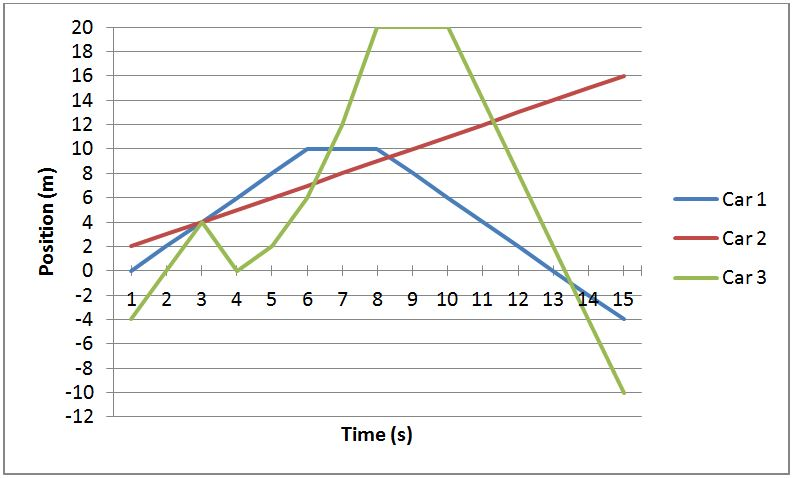At the point where all three cars meet, which car is traveling the fastest?

Car 1.

Car 3.

They are all traveling at the same speed.

Cannot be determined.

Car 2.

Car 3.

Explanation:

The three cars intersect at time = 3s. At this point, one can determine the speed (or velocity) of each car by looking how many meters the car travels per second. At time = 3, car 1 is traveling 1 m per second, car 2 is traveling 2 m per second, and car 3 is traveling 4 m per second. Another way of determining which car is the fastest is by looking at the slopes of each line. The car with the greatest slope is traveling the quickest.

### Example Question #201 : Physics

The graph below depicts the position of three different cars over a 15-second time interval.What is the speed of Car 1 at time = 7s?

1 m/s

4 m/s

2 m/s

Cannot be determined

0 m/s

0 m/s

Explanation:

At time = 7 s, Car 1 holds the same position as it did at 6 and 8 seconds, indicating that the car is not moving. If the car is not moving, then it has a speed of 0/s. One helpful method of determining the speed of a car on this graph, or any similar problem you may encounter, is to look at the slope of the graph. A slope of 0 means the car is not moving. A positive or negative slope indicates the car is in fact moving.

### Example Question #12 : How To Find Data Representation In Physics

The graph below depicts the position of three different cars over a 15-second time interval.If Car 3 continues to travel at it's current speed at t = 15 seconds, what will it's position be at time = 20s?

30 m

–30 m

–26 m

26 m

–34 m

–30 m

Explanation:

At t = 15 s, Car 3 is traveling 4 m/s and is traveling away from position 0 in the negative direction. At t = 15s, Car 3 is also at position = –10 m. Five seconds later it will travel 5s x 4 m/s = 20 m; therefore, it's new position will be (-10 + -20 = -30) –30 m.

### Example Question #11 : How To Find Data Representation In Physics

The graph below depicts the position of three different cars over a 15-second time interval.What is the distance that car 3 has traveled at time = 4s?

4m

8m

–2m

12m

0m

4m

Explanation:

The definition of distance is net change in position. Car 3 begins at position = –4m, and at time = 4s, Car 3 is at 0m. The net change is 4m. In total, car 3 has traveled 12m; however, distance traveled does not account for total amount traveled, but only factors beginning and ending positions.

### Example Question #1321 : Act Science

A physicist wishes to study the trajectory of a ball launched horizontally. She varies parameters such as the launching velocity, starting height, and mass of the ball. For each trajectory, she records the time of flight (in seconds) and horizontal displacement (in meters). She assumes air resistance is negligible.

Figure 1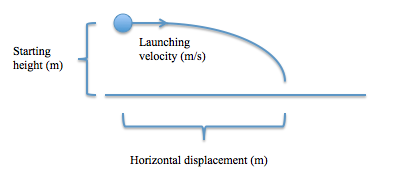Using all of the data she collects, she constructs the following table:

Table 1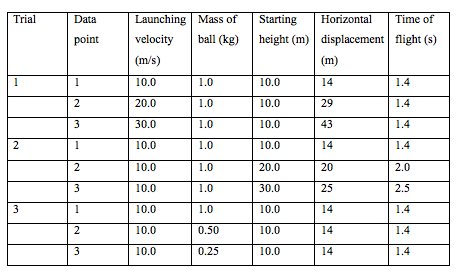In Trial 1, if a fourth data point were collected in which the ball was thrown with a launching velocity of 40 m/s, what would be the approximate horizontal displacement in meters?

63

48

57

52

57

Explanation:

Looking at the table, we see that as the launching velocity is increased by 10 m/s in Trial 1, the horizontal displacement increases by about 14 meters.

### Example Question #12 : How To Find Data Representation In Physics

A physicist wishes to study the trajectory of a ball launched horizontally. She varies parameters such as the launching velocity, starting height, and mass of the ball. For each trajectory, she records the time of flight (in seconds) and horizontal displacement (in meters). She assumes air resistance is negligible.

Figure 1Using all of the data she collects, she constructs the following table:

Table 1If all of trial 3 were redone with a launching velocity of 20 m/s instead of 10 m/s, then compared to the data for trial 3 presented in the table, the horizontal displacement for all data points would be which of the following?

The same

Greater

Smaller

Cannot be determined

Greater

Explanation:

Trial 3 shows us that the mass of the ball does not affect horizontal displacement, so mass can be ignored for this question. Trial 1 shows us that horizontal displacement increases with launching velocity, so we can reason that a higher launching velocity in Trial 3 would result in increased horizontal displacement.

### Example Question #1321 : Act Science

Experiment 1

A scientist develops the following setup, shown in Figure 1 below, to study the charges of radioactive particles. A radioactive sample is placed into a lead box that has an open column such that the particles can only exit from one direction. A detector is placed in front of the opening. A metric ruler (centimeters (cm)), is aligned on the detector such that zero is directly in front of the opening of the column, with the positive values extending to the left and the negative values to the right. On the left side of the experimental setup, there is a device that generates a magnetic field that attracts positively charged particles and repels negatively charged particles.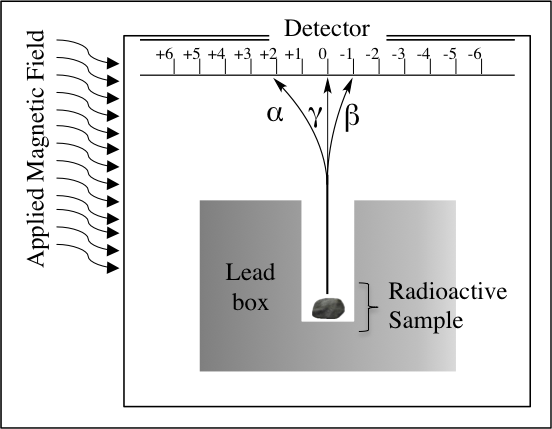Figure 1.

The device detects particles in three different places: alpha, α; beta, β; and gamma, γ; as labeled in Figure 1. The paths these particles take from the source of radioactivity are shown.

Experiment 2

A different scientist finds the following data, shown in Table 1, about the energies of the α, β, and γ particles by observing what kinds of materials through which the particles can pass. This scientist assumes that the ability of particles to pass through thicker and denser barriers is indicative of higher energy. Table 1 summarizes whether or not each type of particle was detected when each of the following barriers is placed between the radioactivity source and the detector. The paper and aluminum foil are both 1 millimeters thick, and the concrete wall is 1 meter thick.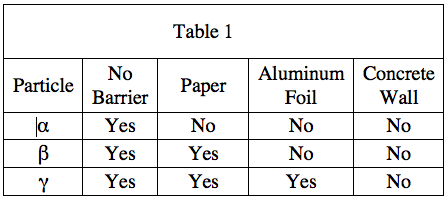Based on Experiment 1, which of the following particles must be positively charged?

α

γ

β

all of the above

α

Explanation:

As the Experiment 1 description states that the magnetic field attracts positively charge particles, and the alpha particle is the only particle with a trajectory bent toward the magnetic field, the answer must be α.

### Example Question #11 : How To Find Data Representation In Physics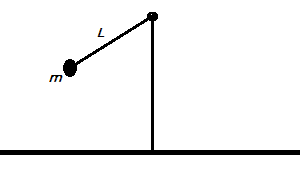The period of a simple pendulum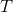is defined as the amount of time that it takes for a pendulum to swing from one end to the other and back. In studying the period of a simple pendulum, two students express their opinions.

Student 1: The period of a pendulum depends on two factors: the mass of the pendulum's bob (the object swinging at the end of the pendulum) and the length of the pendulum. The height at which the pendulum is originally dropped does not affect the period.

Student 2: The period of a pendulumonly depends on the length of the pendulum. Varying the mass and the height at which the pendulum is originally dropped does not affect how long the pendulum takes to swing across.

The two students ran a series of trials to measure the period of a simple pendulum using varying weights and lengths. The students did not measure height as a factor. The results of the trials can be seen in the table below: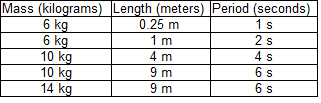According to the data provided, what would we predict would happen if an experiment compared the periods of a pendulum made of a lead weight on a meter-long cord and a pendulum made of a tennis ball on a three-meter-long cord?

The period of the tennis ball pendulum would be shorter than the period of the lead ball pendulum.

The period of the tennis ball pendulum would be longer than the period of the lead ball pendulum.

The period of the tennis ball pendulum would be different than the period of the lead ball pendulum. We do not know whether it would be shorter or longer.

The period of the tennis ball pendulum would be the same as the period of the lead ball pendulum.

The period of the tennis ball pendulum would be longer than the period of the lead ball pendulum.

Explanation:

The table shows in its last two rows that changing the mass of the bob on a pendulum did not affect the pendulum's period. Therefore, only the length of the pendulum appears to affect the period. We can then ignore the lead ball versus the tennis ball, as the mass of each will not affect the periods of their respective pendulums. We can also see that if the length of a pendulum increases, the period of the pendulum also increases. Therefore, we would most likely predict that the period of the longer pendulum would be greater than the period of the shorter pendulum.

### Example Question #12 : How To Find Data Representation In Physics

A scientist is testing the efficiency of a regular heat engine. A heat engine functions in the following manner: a chamber of gas is heated, increasing its pressure, which then causes the gas to expand to relieve some of that pressure. Next, the gas chamber cools off slightly, causing the pressure to decrease once again. This propagates the gas to compress slightly. Simply stated, this process produces mechanical work as the gas's expansion and contraction causes movement. A graph of the heat engine's pressure and volume is represented below, the letters A through D representing steps in the engine's repeating cycle.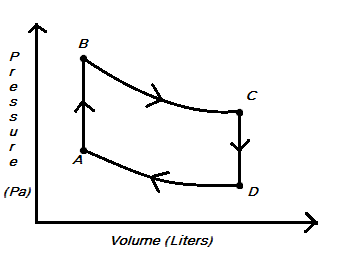Mechanical work for a gas process (movement from one pressure-volume state to another) is calculated using the formula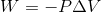, where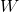represents work,represents pressure (which may or may not be constant), and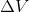represents a change in volume.

According to the equation, in which of the processes in the pressure-volume diagram was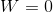?

From D to A

From C to D

From A to B and from C to D.

From B to C

From A to B

From A to B and from C to D.

Explanation:

This question may seem difficult but in reality it is quite easy. Pay attention to the equation given. Sinceis equal to change in volume, a process that involves no change in volume will result in avalue of 0. Plugged into the equation given results in a work value of 0 as well. Therefore, we need to identify any process where the volume did not change. This means A to B and C to D.

### Example Question #13 : How To Find Data Representation In Physics

A scientist is testing the efficiency of a regular heat engine. A heat engine functions in the following manner: a chamber of gas is heated, increasing its pressure, which then causes the gas to expand to relieve some of that pressure. Next, the gas chamber cools off slightly, causing the pressure to decrease once again. This propagates the gas to compress slightly. Simply stated, this process produces mechanical work as the gas's expansion and contraction causes movement. A graph of the heat engine's pressure and volume is represented below, the letters A through D representing steps in the engine's repeating cycle.Mechanical work for a gas process (movement from one pressure-volume state to another) is calculated using the formula, whererepresents work,represents pressure (which may or may not be constant), andrepresents a change in volume.

A gas process is considered isobaric if the pressure is held constant throughout the process. Which processes involved in the heat engine cycle described above can be considered isobaric?

None of the above processes were isobaric.

B to C

C to D

D to A

A to B

An isobaric process means that pressure does not change throughout the process. If you look at the pressure volume diagram provided, pressure is always changing. You just need to follow the process along with your eye and see that a movement from any point in the cycle to another involves either moving up or down the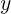-axis. This shows you that pressure is always changing in this cycle. This is one reason why pressure volume diagrams are so useful when describing engines!